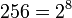# SmallGroup(256,27799)

View a complete list of particular groups (this is a very huge list!)[SHOW MORE]

## Definition

This is a group of order$256 = 2^8$ given by the following presentation:$\langle a_1, a_2, a_3, a_4, a_5 \mid a_1^2 = a_2^2 = a_3^4 = a_4^4 = a_5^4 = e, [a_1,a_2] = a_3^2, [a_1,a_3] = e, [a_2,a_3] = a_4^2, [a_1,a_4] = [a_2,a_4] = e, [a_3,a_4] = a_5^2, [a_1,a_5] = [a_2,a_5] = [a_3,a_5] = [a_4,a_5] = e \rangle$

Here$e$ denotes the identity element and$[ \ , \ ]$ stands for the commutator of two elements. It does not matter whether we use the left or right action convention -- although the specific words are different in both conventions, the groups defined are isomorphic.

## GAP implementation

### Group ID

This finite group has order 256 and has ID 27799 among the groups of order 256 in GAP's SmallGroup library. For context, there are groups of order 256. It can thus be defined using GAP's SmallGroup function as:

SmallGroup(256,27799)

For instance, we can use the following assignment in GAP to create the group and name it$G$:

gap> G := SmallGroup(256,27799);

Conversely, to check whether a given group$G$ is in fact the group we want, we can use GAP's IdGroup function:

IdGroup(G) = [256,27799]

or just do:

IdGroup(G)

to have GAP output the group ID, that we can then compare to what we want.

### Description by presentation

gap> F := FreeGroup(5);
<free group on the generators [ f1, f2, f3, f4, f5 ]>
gap> R1 := [F.1^2,F.2^2,F.3^4,F.4^4,F.5^4];
[ f1^2, f2^2, f3^4, f4^4, f5^4 ]
gap> R2 := [Comm(F.1,F.2)*F.3^(-2)];
[ f1^-1*f2^-1*f1*f2*f3^-2 ]
gap> R3 := [Comm(F.1,F.3),Comm(F.2,F.3)*F.4^(-2)];
[ f1^-1*f3^-1*f1*f3, f2^-1*f3^-1*f2*f3*f4^-2 ]
gap> R4 := [Comm(F.1,F.4),Comm(F.2,F.4),Comm(F.3,F.4)*F.5^(-2)];
[ f1^-1*f4^-1*f1*f4, f2^-1*f4^-1*f2*f4, f3^-1*f4^-1*f3*f4*f5^-2 ]
gap> R5 := [Comm(F.1,F.5),Comm(F.2,F.5),Comm(F.3,F.5),Comm(F.4,F.5)];
[ f1^-1*f5^-1*f1*f5, f2^-1*f5^-1*f2*f5, f3^-1*f5^-1*f3*f5, f4^-1*f5^-1*f4*f5 ]
gap> R := Union([R1,R2,R3,R4,R5]);
[ f1^2, f2^2, f1^-1*f3^-1*f1*f3, f1^-1*f4^-1*f1*f4, f1^-1*f5^-1*f1*f5, f2^-1*f4^-1*f2*f4, f2^-1*f5^-1*f2*f5, f3^-1*f5^-1*f3*f5, f3^4, f4^-1*f5^-1*f4*f5,
f4^4, f5^4, f1^-1*f2^-1*f1*f2*f3^-2, f2^-1*f3^-1*f2*f3*f4^-2, f3^-1*f4^-1*f3*f4*f5^-2 ]
gap> G := F/R;
<fp group on the generators [ f1, f2, f3, f4, f5 ]>